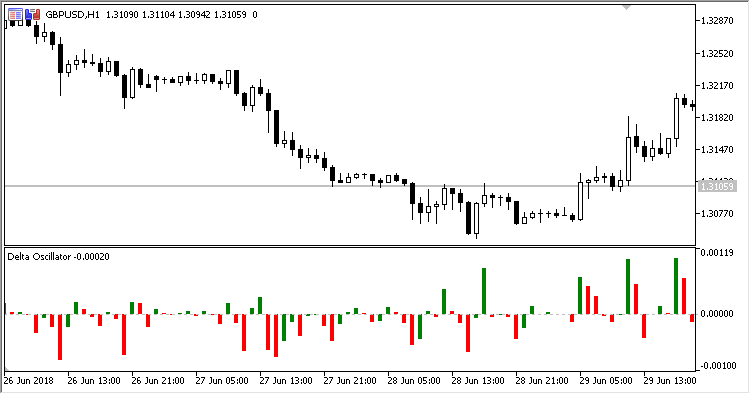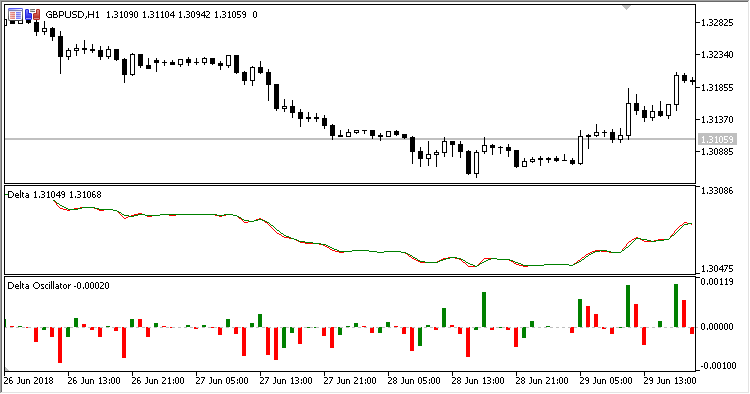Interesting script?
So post a link to it -
let others appraise it

You liked the script? Try it in the MetaTrader 5 terminal# Delta_Oscillator - indicator for MetaTrader 5

Views:
1123
Rating:
Published:
2018.09.27 15:28

The Delta Oscillator indicator displays the difference in the Delta indicator lines as a histogram.

The indicator has no input parameters.

Calculations:

`OSC = Price - Delta`

where:

```Delta = Price + Diff
Diff = Log10(PrevPrice / Price)
Price = (Open + High + Low + Close) / 4.0
```Fig. 1. Delta_oscillatorFig. 2. Delta_oscillator + Delta indicator

Translated from Russian by MetaQuotes Software Corp.
Original code: https://www.mql5.com/ru/code/21712Delta

The Delta indicator draws two lines: the bar average price line (OHLC)/4 and the line of the decimal logarithm of the current to previous (OHLC)/4 prices ratio.Darvas_Box

A channel filter indicator Darvas Box.Deviation_Oscillator

Deviation Oscillator - a volatility oscillator which represents a difference between the price and the MA, normalized within the selected range.DHLBO

The DHLBO (Dynamic High/Low Band Overlay) indicator shows on the price chart two bands calculated based on the range Highs/Lows.